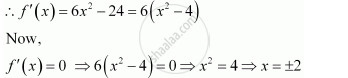Share

# Find the Maximum Value of 2x3 − 24x + 107 in the Interval [1, 3]. Find the Maximum Value of the Same Function in [−3, −1]. - Mathematics

#### Question

Find the maximum value of 2x3 − 24x + 107 in the interval [1, 3]. Find the maximum value of the same function in [−3, −1].

#### Solution

Let f(x) = 2x3 − 24x + 107.We first consider the interval [1, 3].

Then, we evaluate the value of f at the critical point x = 2 ∈ [1, 3] and at the end points of the interval [1, 3].

f(2) = 2(8) − 24(2) + 107 = 16 − 48 + 107 = 75

f(1) = 2(1) − 24(1) + 107 = 2 − 24 + 107 = 85

f(3) = 2(27) − 24(3) + 107 = 54 − 72 + 107 = 89

Hence, the absolute maximum value of f(x) in the interval [1, 3] is 89 occurring at x = 3.

Next, we consider the interval [−3, −1].

Evaluate the value of f at the critical point x = −2 ∈ [−3, −1] and at the end points of the interval [1, 3].

f(−3) = 2 (−27) − 24(−3) + 107 = −54 + 72 + 107 = 125

f(−1) = 2(−1) − 24 (−1) + 107 = −2 + 24 + 107 = 129

f(−2) = 2(−8) − 24 (−2) + 107 = −16 + 48 + 107 = 139

Hence, the absolute maximum value of f(x) in the interval [−3, −1] is 139 occurring at x = −2.

Is there an error in this question or solution?